## Contents

 |   Tiled Menu   |   Tables of Content   |  Banner Video   |  Tohline Home Page   |

[Preamble by Joel E. Tohline — Circa 2020]  In an effort to serve ongoing research activities of the astrophysics community, this media-wiki-based hypertext book (H_Book) reviews what is presently understood about the structure, stability, and dynamical evolution of (Newtonian) self-gravitating fluids. In the context of this review, the "dynamical evolution" categorization generally will refer to studies that begin with an equilibrium configuration that has been identified — via a stability analysis — as being dynamically (or, perhaps, secularly) unstable then, using numerical hydrodynamic technique, follow the development to nonlinear amplitude of deformations that spontaneously develop as a result of the identified instability.

As is reflected in our choice of the overarching set of Principle Governing Equations, our focus is on studies of compressible fluid systems — those that obey a barotropic equation of state. But, in addition, chapters are included that review what is known about the structure and stability of self-gravitating incompressible (uniform-density) fluid systems because: (a) in this special case, the set of governing relations is often amenable to a closed-form, analytic solution; and (b) most modern computationally assisted studies of compressible fluid systems — both in terms of their design and in the manner in which results have been interpreted — have been heavily influenced by these, more classical, studies of incompressible fluid systems.

As the layout of the following tiled menu reflects, this review is broken into three major topical areas based primarily on geometrical considerations:

• Studies of configurations that are — at least initially — spherically symmetric.
• Two-dimensional configurations — such as rotationally flattened spheroidal-like or toroidal-like structures; or infinitesimally thin, but nonaxisymmetric, disk-like structures.
• Configurations that require a full three-dimensional treatment — most notably, "spinning" ellipsoidal-like structures; or binary systems.

This is very much a living review. The chosen theme encompasses an enormous field of research that, because of its relevance to the astrophysics community, over time is continuing to expand at a healthy pace. As a consequence the review is incomplete now, and it will always be incomplete, so please bear with me. On any given day/week/month I will turn my attention to a topic that seems particularly interesting to me and I will begin writing a new chapter or I will edit/expand the contents of an existing tiled_menu chapter. This necessarily means that all chapters are incomplete while, in practice, some are much more polished than others. Hopefully steady forward progress is being made and the review will indeed be viewed as providing a service to the community.

## Spherically Symmetric Configurations

(Initially) Spherically Symmetric Configurations

### Equilibrium Structures

1D STRUCTURE

Hydrostatic
Balance
Equation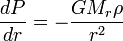$~\frac{dP}{dr} = - \frac{GM_r \rho}{r^2}$
Solution
Strategies

Isothermal
Sphere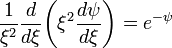$~\frac{1}{\xi^2} \frac{d}{d\xi}\biggl( \xi^2 \frac{d\psi}{d\xi} \biggr) = e^{-\psi}$
via
Direct
Numerical
Integration

Isolated
Polytropes
Lane
(1870)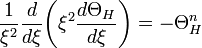$~\frac{1}{\xi^2} \frac{d}{d\xi}\biggl( \xi^2 \frac{d\Theta_H}{d\xi} \biggr) = - \Theta_H^n$
Known
Analytic
Solutions
via
Direct
Numerical
Integration
via
Self-Consistent
Field (SCF)
Technique

Free Energy
of
Bipolytropes

(nc, ne) = (5, 1)

### Stability Analysis

1D STABILITY

Pressure-Truncated
Isothermal
Spheres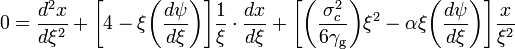$~0 = \frac{d^2x}{d\xi^2} + \biggl[4 - \xi \biggl( \frac{d\psi}{d\xi} \biggr) \biggr] \frac{1}{\xi} \cdot \frac{dx}{d\xi} + \biggl[ \biggl( \frac{\sigma_c^2}{6\gamma_\mathrm{g}}\biggr)\xi^2 - \alpha \xi \biggl( \frac{d\psi}{d\xi} \biggr) \biggr] \frac{x}{\xi^2}$ where: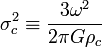$~\sigma_c^2 \equiv \frac{3\omega^2}{2\pi G\rho_c}$     and,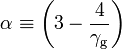$~\alpha \equiv \biggl(3 - \frac{4}{\gamma_\mathrm{g}}\biggr)$
via
Direct
Numerical
Integration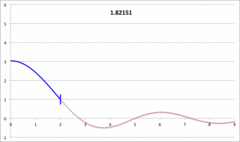Yabushita's
Analytic Sol'n
for
Marginally Unstable
Configurations
(1974)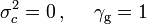$~\sigma_c^2 = 0 \, , ~~~~\gamma_\mathrm{g} = 1$ and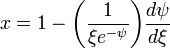$~x = 1 - \biggl( \frac{1}{\xi e^{-\psi}}\biggr) \frac{d\psi}{d\xi}$

Polytropes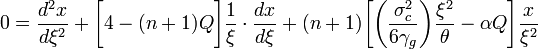$~0 = \frac{d^2x}{d\xi^2} + \biggl[ 4 - (n+1) Q \biggr] \frac{1}{\xi} \cdot \frac{dx}{d\xi} + (n+1) \biggl[ \biggl( \frac{\sigma_c^2}{6\gamma_g } \biggr) \frac{\xi^2}{\theta} - \alpha Q\biggr] \frac{x}{\xi^2}$ where: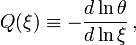$~Q(\xi) \equiv - \frac{d\ln\theta}{d\ln\xi} \, ,$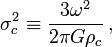$~\sigma_c^2 \equiv \frac{3\omega^2}{2\pi G\rho_c} \, ,$     and,$~\alpha \equiv \biggl(3 - \frac{4}{\gamma_\mathrm{g}}\biggr)$
Isolated
n = 3
Polytrope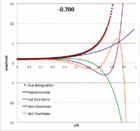Pressure-Truncated
n = 5
Configurations

Our Analytic Sol'n
for
Marginally Unstable
Configurations
(2017)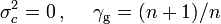$~\sigma_c^2 = 0 \, , ~~~~\gamma_\mathrm{g} = (n+1)/n$ and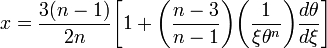$~x = \frac{3(n-1)}{2n}\biggl[1 + \biggl(\frac{n-3}{n-1}\biggr) \biggl( \frac{1}{\xi \theta^{n}}\biggr) \frac{d\theta}{d\xi}\biggr]$

1D DYNAMICS

## Two-Dimensional Configurations (Axisymmetric)

(Initially) Axisymmetric Configurations

### Axisymmetric Equilibrium Structures

2D STRUCTURE

#### Toroidal & Toroidal-Like

Definition: anchor ring

### Stability Analysis

2D STABILITY

#### Sheroidal & Spheroidal-Like

 The equilibrium models are calculated using the polytrope version (Bodenheimer & Ostriker 1973) of the Ostriker and Mark (1968) self-consistent field (SCF) code … the equilibrium models rotate on cylinders and are completely specified by$~n$, the total angular momentum, and the specific angular momentum distribution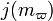$~j(m_\varpi)$. Here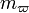$~m_\varpi$ is the mass contained within a cylinder of radius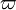$~\varpi$ centered on the rotation axis. The angular momentum distribution is prescribed in several ways: (1) imposing strict uniform rotation; (2) using the same$~j(m_\varpi)$ as that of a uniformly rotating spherical polybrope of index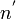$~n^'$ (see Bodenheimer and Ostriker 1973); and (3) using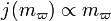$~j(m_\varpi) \propto m_\varpi$, which we refer to as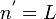$~n^' = L$,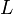$~L$ for "linear."

2D DYNAMICS

## Two-Dimensional Configurations (Nonaxisymmetric Disks)

Infinitesimally Thin, Nonaxisymmetric Disks

2D STRUCTURE

## Three-Dimensional Configurations

(Initially) Three-Dimensional Configurations

### Equilibrium Structures

3D STRUCTURE
 "One interesting aspect of our models … is the pulsation characteristic of the final central triaxial figure … our interest in the pulsations stems from a general concern about the equilibrium structure of self-gravitating, triaxial objects. In the past, attempts to construct hydrostatic models of any equilibrium, triaxial structure having both a high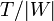$~T/|W|$ value and a compressible equation of state have met with very limited success … they have been thwarted by a lack of understanding of how to represent complex internal motions in a physically realistic way… We suggest … that a natural attribute of [such] configurations may be pulsation and that, as a result, a search for simple circulation hydrostatic analogs of such systems may prove to a fruitless endeavor. — Drawn from §IVa of Williams & Tohline (1988), ApJ, 334, 449
 Special numerical techniques must be developed "to build three-dimensional compressible equilibrium models with complicated flows." To date … "techniques have only been developed to build compressible equilibrium models of nonaxisymmetric configurations for a few systems with simplified rotational profiles, e.g., rigidly rotating systems (Hachisu & Eriguchi 1984; Hachisu 1986), irrotational systems (Uryū & Eriguchi 1998), and configurations that are stationary in the inertial frame (Uryū & Eriguchi 1996)." — Drawn from §1 of Ou (2006), ApJ, 639, 549

#### Binary Systems

• S. Chandrasekhar (1933), MNRAS, 93, 539: The equilibrium of distorted polytropes. IV. the rotational and the tidal distortions as functions of the density distribution
• S. Chandrasekhar (1963), ApJ, 138, 1182: The Equilibrium and the Stability of the Roche Ellipsoids
 Roche's problem is concerned with the equilibrium and the stability of rotating homogeneous masses which are, further, distorted by the constant tidal action of an attendant rigid spherical mass.

3D STABILITY

3D DYNAMICS